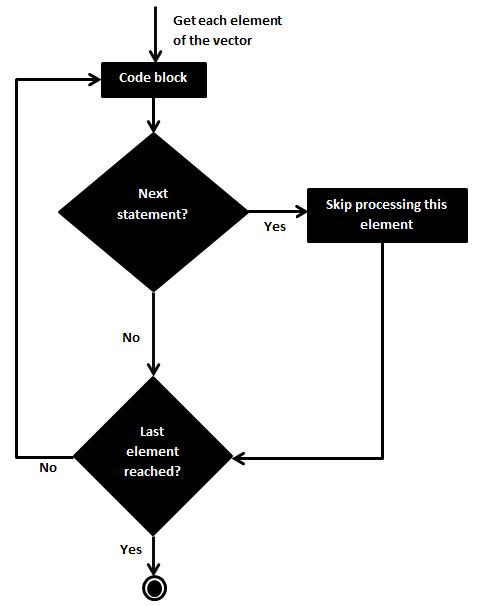# R - Next Statement

The next statement in R programming language is useful when we want to skip the current iteration of a loop without terminating it. On encountering next, the R parser skips further evaluation and starts next iteration of the loop.

## Syntax

The basic syntax for creating a next statement in R is −

```next
```

## Flow Diagram## Example

```v <- LETTERS[1:6]
for ( i in v) {

if (i == "D") {
next
}
print(i)
}
```

When the above code is compiled and executed, it produces the following result −

``` "A"
 "B"
 "C"
 "E"
 "F"
```
r_loops.htm